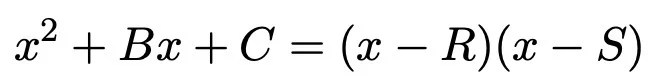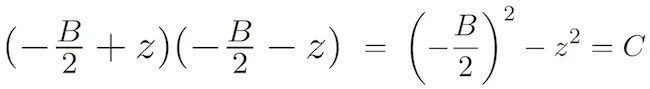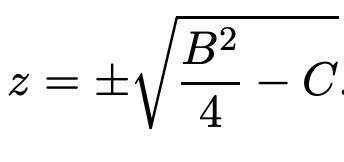# Simplest Way To Solve Quadratic Equations Has Been Overlooked For 4,000 Years

• A math professor discovered a new way to solve quadratic equations.
• It’s quite surprising that such a simple method gas gone unnoticed for 4,000 years.

In elementary algebra, quadratic equations can be solved by using different methods, such as factoring, graphing, completing the square, and others.

The history of quadratic formula — a formula provides the solution(s) to a quadratic equation — can be traced back to the Old Babylonian Period around 2000–1600 B.C. Many great mathematicians left their mark on the subject, and the formula became one of the most important parts in algebra.

The formula, however, is quite tricky and its computations are somewhat messy. It could be challenging for first-time Algebra learners to follow.

Recently, a mathematician at Carnegie Mellon University in Pittsburgh, published a simpler to solve any quadratic equation. This new method is easy-to-remember and computationally-efficient.

According to its author, Po-Shen Loh, it has the potential to demystify the quadratic formula for students all across the world.

### Alternative Method To Solve Quadratics

The very first step is to see if a quadratic equation can be factorized in the following way:If factorization is possible, the quadratic function is zero when X=R or X=S. As per the traditional method, if the sum and product of R and S is -B and C, respectively, then {R, S} will be the complete set of roots.

Now here comes the twist.

Two numbers sum to -B precisely when their average is -B/2. Let’s consider these two numbers in the form of -B/2 ± z, where z is an unknown quantity, and the product of these numbers is equal to C.If z turns out to be zero, then we factor with R=S=(-B/2), otherwise,Reference: arXiv:1910.06709

The square root always exists (taking complex numbers into account), which is why the desired R and S always exist for any quadratic equation. Thus, the original roots can be expressed asAnd that’s the new quadratic formula; much simpler and easy to remember than the previous one.

### Why Now?

The new method is intuitive and it does not require the formula to be memorized at all. However, the more interesting question is why no one has thought of it before.

The author has searched the 4,000 years of history on this topic: he studied various approaches built by the ancient Babylonians, Greeks, Indians, Arabs, and Chinese as well as modern mathematicians, but didn’t find anything that resembles his method.

Po-Shen Loh thinks it’s related to the way the traditional method proves that quadratic equations have two roots. It is generally considered that a quadratic equation always has two roots, and those roots have product C and -B.

Perhaps, by the time mathematics had advanced to a ‘decent level’, the Babylonian technique had faded out of recent memory, and people found the approach of completing the square to be good enough to integrate into the mainstream curriculum.

Now the question is how quickly and how widely it will spread.Written by
###### Varun Kumar

Varun Kumar is a professional science and technology journalist and a big fan of AI, machines, and space exploration. He received a Master's degree in computer science from GGSIPU University. To find out about his latest projects, feel free to directly email him at [email protected]